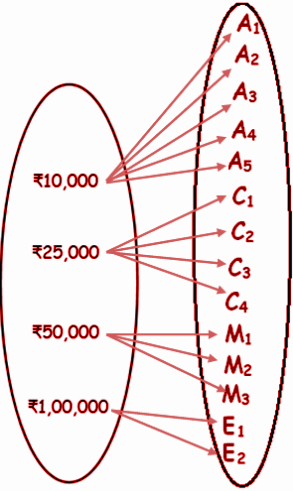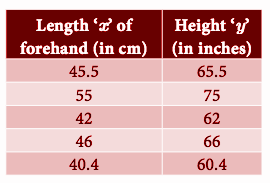# Samacheer Kalvi 10th Maths Book Solutions Chapter 1 | Relations and Functions Answers For Tamil Nadu Board

Samacheer Kalvi 10th Maths Book Solutions Chapter 1 – Relations and Functions are available here. The Samacheer Kalvi 10th Maths book answers of Chapter 1, available at BYJU’S, contain step by step explanations designed by our Mathematics experts. All these important questions are based on the new pattern prescribed by the Tamil Nadu board. Students can also get the solutions of other chapters on Samacheer Kalvi 10th Maths solutions. Referring to the Samacheer Kalvi Class 10 Maths Book Chapter 1  Solutions is the best way to gauge the performance, after solving these questions from the textbook.

Chapter 1 of the Samacheer Kalvi 10th Maths guide will help the students to solve problems related to the ordered pair, cartesian product, relations, functions, representation of functions, vertical line test, types of functions, horizontal line test, special cases of functions, the composition of functions, identifying the graphs of linear, quadratic, cubic and reciprocal functions.

### Samacheer Kalvi 10th Maths Chapter 1: Relations and Functions Book Exercise 1.1 Questions and Solutions

Question 1: Let A = {1, 2, 3} and B = {x | x is a prime number less than 10}. Find A x B and B x A.

Solution:

A = {1, 2, 3}

B = {x | x is a prime number less than 10}.

B = {2, 3, 5, 7}

A x B = { (1, 2) (1, 3) (1, 5) (1, 7)(2, 2) (2, 3) (2, 5) (2, 7)(3, 2) (3, 3) (3, 5) (3, 7) }

B x A = { (2, 1) (2, 2) (2, 3)(3, 1) (3, 2) (3, 3) (5, 1) (5, 2) (5, 3) (7, 1) (7, 2) (7, 3) }

Question 2: Let A = {x ∈ W | x < 2} , B = {x ∈|1 < x ≤ 4} and C = {3, 5} . Verify that

(i) A × (B U C) = (A × B) U (A × C)

(ii) A × (B n C) = (A × B) n (A × C)

(iii) (A U B) × C = (A × C) U (B × C)

Solution:

A = {x ∈ W | x < 2} , B = {x ∈|1 < x ≤ 4} and C = {3, 5} .

A = {0, 1} , B = {2, 3, 4} and C = {3, 5} .

(i) A × (B U C) = (A × B) U (A × C)

Consider the LHS

(B U C) = {2, 3, 4, 5}

A × (B U C)

= {(0, 2) (0, 3) (0, 4) (0, 5)(1, 2) (1, 3) (1, 4) (1, 5)} ——-(1)

Take the RHS

(A × B) = {(0, 2) (0, 3) (0, 4)(1, 2) (1, 3) (1, 4)}

(A × C) = {(0, 3)(0, 5) (1, 3) (1, 5)}

(A x B) U (A x C) = {(0, 2) (0, 3) (0, 4) (0, 5)(1, 2) (1, 3) (1, 4) (1, 5)} ——(2)

(1) = (2)

(ii) A × (B n C) = (A × B) n (A × C)

A = {0, 1} , B = {2, 3, 4} and C = {3, 5} .

Consider the LHS

(B n C) = {3}

A × (B n C) = {(0, 3) (1, 3)} ——(1)

Take the RHS

(A × B) = {(0, 2) (0, 3) (0, 4)(1, 2) (1, 3) (1, 4)}

(A × C) = {(0, 3)(0, 5) (1, 3) (1, 5)}

(A × B) n (A × C) = {(0, 3) (1, 3)} ——(2)

(1) = (2)

(iii) (A U B) × C = (A × C) U (B × C)

A = {0, 1} , B = {2, 3, 4} and C = {3, 5} .

A U B = {0, 1, 2, 3, 4}

(A U B) × C = { (0, 3)(0, 5)(1, 3)(1, 5)(2, 3)(2, 5)(3, 3)(3, 5)(4, 3)(4, 5) } —–(1)

(A × B) = {(0, 2) (0, 3) (0, 4)(1, 2) (1, 3) (1, 4)}

(B × C) = {(2, 3) (2, 5) (3, 3)(3, 5) (4, 3) (4, 5)}

(A × C) U (B × C) = { (0, 3)(0, 5)(1, 3)(1, 5)(2, 3)(2, 5)(3, 3)(3, 5)(4, 3)(4, 5) } —–(2)

(1) = (2)

### Samacheer Kalvi 10th Maths Chapter 1: Relations and Functions Book Exercise 1.2 Questions and Solutions

Question 1: Let A = {1, 2, 3, 4,…, 45} and R be the relation defined as “is square of ” on A. Write R as a subset of A x A. Also, find the domain and range of R.

Solution:

A = {1, 2, 3, 4,…, 45}

Let “A” be a set which contains some of the elements of A

A = {1, 2, 3, 4, 5, 6}

Now, write the other set of elements from A. The elements in this set denotes the square value of the previous set.

Square values of set A = {1, 4, 9, 16, 25, 36}

A x A = {(1, 1)(1,4)(1, 9)(1, 16) (1, 25) (1, 36)………………}

Domain = {1, 2, 3, 4, 5, 6}

Range = {1, 4, 9, 16, 25, 36}

Question 2: A company has four categories of employees given by Assistants (A), Clerks (C), Managers (M) and an Executive Officer (E). The company provides ₹10,000, ₹25,000, ₹50,000 and ₹1,00,000 as salaries to the people who work in the categories A, C, M and E, respectively. If A1, A2, A3, A4 and A5 were Assistants; C1, C2, C3, C4 were Clerks; M1, M2, M3 were managers and E1, E2 was Executive officers and if the relation R is defined by xRy, where x is the salary given to person y, express the relation R through an ordered pair and an arrow diagram.

Solution:

Let x be the salary given to the person.

Let y be the set of employees.### Samacheer Kalvi 10th Maths Chapter 1: Relations and Functions Book Exercise 1.3 Questions and Solutions

Question 1: A function f is defined by f (x) = 2x – 3

(i) find [f (0) + f (1)] / 2

(ii) find x such that f (x) = 0.

(iii) find x such that f (x) = x .

(iv) find x such that f (x) = f (1−x) .

Solution:

f(x) = 2x – 3

(i) [f(0) + f(1)] / 2

f(0) = 2(0) – 3 = -3

f(1) = 2(1) – 3 = -1

[f(0) + f(1)] / 2 = [-3 + (-1)] / 2

= -4 / 2

= -2

(ii) find x such that f (x) = 0.

f(x) = 2x – 3

2x – 3 = 0

2x = 3

x = 3 / 2

(iii) find x such that f (x) = x .

f(x) = 2x – 3

2x – 3 = x

2x – x = 3

x = 3

(iv) find x such that f (x) = f (1−x) .

f(1 – x) = 2(1- x) – 3

= 2 – 2x – 3

= -2x – 1

2x – 3 = -2x – 1

2x + 2x = – 1 + 3

4x = 2

x = 2 / 4 = 1 / 2

Question 2: The data in the adjacent table depicts the length of a woman’s forehand and her corresponding height. Based on this data, a student finds a relationship between the height (y) and the forehand length(x) as y = ax +b, where a, b are constants.(i) Check if this relation is a function.

(ii) Find a and b.

(iii) Find the height of a woman whose forehand length is 40 cm.

(iv) Find the length of the forehand of a woman if her height is 53.3 inches.

Solution:

y = ax + b

(i) For every value of x, different values of y are obtained. So, it is a function.

(ii) y = a x + b

x = 45.5, y = 65.5

65.5 = a(45.5) + b

65.5 = 45.5 a + b –(1)

y = a x + b

x = 35, y = 56

56 = a(35) + b

56 = 35 a + b –(2)

(1) – (2)

(45.5 a + b) – (35a + b) = 65.5 – 56

45.5 a – 35a + b – b = 9.5

10.5a = 9.5

a = 9.5 / 10.5

a = 0.90

Substitute a = 0.90 in (1),

45.5(0.90) + b = 65.5

b = 65.5 – 40.95

b = 24.5

y = 0.9x + 24.5

(iii) Find the height of a woman whose forehand length is 40 cm.

y = ? if x = 40

y = 0.9(40) + 24.5

y = 60.5

Height of the woman is 60.5 inches.

(iv) x = ? if y = 53.3

53.3 = 0.9x + 24.5

53.3 – 24.5 = 0.9x

28.8 / 0.9 = x

x = 32

Length of the forehand is 32 inches.

### Samacheer Kalvi 10th Maths Chapter 1: Relations and Functions Book Exercise 1.4 Questions and Solutions

Question 1: Show that the function f : N -> N defined by f (x) = 2x – 1 is one-one but not onto.

Solution:

If for all a1, a2 ∈ A, f(a1) = f(a2) implies a1 = a2, then f is called one – one function.

Let x, y ∈ N, f(x) = f(y)

f(x) = 2x – 1 —–(1)

f(y) = 2y – 1 —–(2)

(1) = (2)

2x – 1 = 2y – 1

2x = 2y

x = y

Hence,+ the function is one to one.

It is not onto :

If the codomain of the function is equal to the range of function, then the function is onto.

Even numbers in the codomain are not associated with the elements of the domain.

So, it is not onto.

Question 2: Show that the function f : N -> N defined by f (m) = m2 + m + 3 is a one-one function.

Solution:

Let x, y ∈ N, f(x) = f(y)

f (m) = m2 + m + 3

f(x) = x2 + x + 3 —–(1)

f(y) = y2 + y + 3 —–(2)

(1) = (2)

x2 + x + 3 = y2 + y + 3

x2 + x = y2 + y

x2 – y2 + x – y = 0

(x + y) (x – y) + (x – y) = 0

(x – y) (x + y + 1) = 0

x – y = 0

x = y

Hence, it is one to one function.

### Samacheer Kalvi 10th Maths Chapter 1: Relations and Functions Book Exercise 1.5 Questions and Solutions

Question 1: Let f = {(−1, 3),(0,−1),(2,−9)} be a linear function from Z into Z . Find f (x).

Solution:

Let the linear function be y = ax + b.

If x = -1, then y = 3

3 = a(-1) + b

3 = -a + b

-a + b = 3 ——-(1)

If x = 0, then y = -1

-1 = a(0) + b

-1 = b

b = -1

By applying the value of b in (1)

-a + (-1) = 3

-a = 3 + 1

-a = 4

a = -4

Substitute the values of a and b,

y = -4x – 1

The linear equation is -4x – 1.

Question 2: In electrical circuit theory, a circuit C(t) is called a linear circuit if it satisfies the superposition principle given by C(at1 + bt2) = aC(t1) + bC(t2), where a, b are constants. Show that the circuit C(t) = 3t is linear.

Solution:

Let the two points t1 and t2 be taken as the domain of C(t).

c(at1 + bt2) = 3(at1 + bt2)

c(at1) = 3at1

ac(t1) = 3at1

bc(t2) = 3bt2

3(at1 + bt2) = 3at1 + 3at2

C(at1 + bt2) = aC(t1) + bC(t2),

Hence, c(t) is linear.